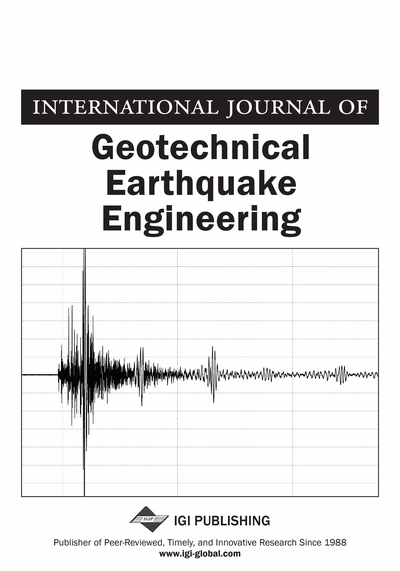# SPT-Based Probabilistic Method for Evaluation of Liquefaction Potential of Soil Using Multi-Gene Genetic Programming

Pradyut Kumar Muduli (Department of Civil Engineering, National Institute of Technology, Rourkela, Odisha, India) and Sarat Kumar Das (Department of Civil Engineering, National Institute of Technology, Rourkela, Odisha, India)
DOI: 10.4018/jgee.2013010103

## Abstract

The present study discusses about evaluation of liquefaction potential of soil within a probabilistic framework based on the standard penetration test (SPT) dataset using evolutionary artificial intelligence technique, multi-gene genetic programming (MGGP). Based on the developed limit state function, a relationship is given between probability of liquefaction and factor of safety against liquefaction using Bayesian theory. This Bayesian mapping function is further used to develop a probabiliy based design chart for evaluation of liquefaction potential of soil. Using an independent database the efficacy of present MGGP based probabilistic model is compared with the available artificial neural network (ANN) and statistical models in terms of rate of successful prediction of liquefaction and non-liquefaction cases. The proposed MGGP based model is found to be more accurate compared to other models.
Article Preview
Top

## 1. Introduction

Soil liquefaction caused large scale damage to infrastructures and life line systems during many historical earthquakes. Though different approaches like cyclic strain based, energy based and cyclic stress based approach are in use, the stress based approach using the in-situ data is the most widely used method for evaluation of liquefaction potential of soil (Krammer, 1996). National Center for Earthquake Engineering Research (NCEER) workshop, 1998, published the reviews of in-situ test based simplified methods (Seed & Idriss, 1971; Seed et al., 1985) for evaluation of liquefaction potential of soil (Youd et al., 2001). The discussed methods are deterministic methods, in which liquefaction potential of soil is evaluated in terms of factor of safety against liquefaction (Fs), defined as the ratio of cyclic resistance ratio (CRR) to cyclic stress ratio (CSR). However, due to parameter and model uncertainties, Fs>1 may not always indicate non-liquefaction case and similarly Fs≤1 may not always correspond to liquefaction (Juang et al., 2000). In order to overcome the mentioned difficulties in deterministic approach, probabilistic evaluation of liquefaction potential has been performed where liquefaction potential is expressed in terms of probability of liquefaction. The boundary curve that separates liquefaction and non-liquefaction cases in the deterministic methods is considered as performance function or “limit state function” (Juang et al., 2000) and is generally biased towards conservative side by encompassing most of the liquefied cases. But, the degree of conservatism is not quantified.

Thus, attempts have been made by several researchers to quantify the unknown degree of conservatism associated with the limit state function and to assess liquefaction potential in terms of probability of liquefaction (PL) using statistical or probabilistic approaches. Halder and Tang (1979) applied first order second moment (FOSM) method of reliability analysis to the method proposed by Seed and Idriss (1971) to estimate the probability of liquefaction. Lio et al. (1988), Youd & Nobble (1997), and Toprak et al. (1999) used logistic regression analyses of post liquefaction field performance data to develop empirical equations for assessing probability of liquefaction. But, in geotechnical engineering practice the design decisions are generally based on deterministic approach.-Juang et al. (2000) proposed a Bayesian mapping function based on SPT dataset to relate Fs with PL. Cetin (2000) and Cetin et al. (2004) developed SPT based probabilistic models for evaluation of liquefaction potential using first order reliability method (FORM) and a Bayesian updating technique.

Artificial intelligence (AI) techniques; artificial neural network (ANN) (Goh, 1994; Juang et al., 2000; Hanna et al., 2007; Samui & Sitharam, 2011), support vector machine (SVM) (Pal, 2006; Goh & Goh, 2007; Samui & Sitharam, 2011) and relevance vector machine (RVM) (Samui, 2007) have been used to develop liquefaction prediction models, based on in-situ test database, which are found to be more efficient compared to statistical methods. However, the ANN is known as ‘black box’ system unable to comprehensively explain the input to output relationship and also needs iterative learning steps to obtain better generalization performances. Though, SVM has better generalization compared to ANN, but the parameters ‘C’ and insensitive loss function (ε) needs to be fine tuned by the user. Moreover, the model equations as per ANN and SVM (Das et al., 2011) are not compact and convenient for its further application.

## Complete Article List

Search this Journal:
Reset
Open Access Articles: Forthcoming
Volume 12: 2 Issues (2021): Forthcoming, Available for Pre-Order
Volume 11: 2 Issues (2020): 1 Released, 1 Forthcoming
Volume 10: 2 Issues (2019)
Volume 9: 2 Issues (2018)
Volume 8: 2 Issues (2017)
Volume 7: 2 Issues (2016)
Volume 6: 2 Issues (2015)
Volume 5: 2 Issues (2014)
Volume 4: 2 Issues (2013)
Volume 3: 2 Issues (2012)
Volume 2: 2 Issues (2011)
Volume 1: 2 Issues (2010)
View Complete Journal Contents Listing